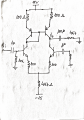# Differential Pair with Active Load Problem

#### dacarvalho

Joined Oct 27, 2017
12
Hello dudes,

I need some help with this differential pair circuit, i'm frustrated because i dont even know how to start the calculations. The circuit is this:So we need to do some previsions in order to confirm the results on practical class.
We have the following questions. Calculate:
1. Differential Gain of circuit
2. Common mode gain
3. Cutoff frequencies of circuit

#### Jony130

Joined Feb 17, 2009
5,243
Are you sure that your circuit diagram does not contain any error?
As for where to start. First, try to find the DC current.

#### dacarvalho

Joined Oct 27, 2017
12
Are you sure that your circuit diagram does not contain any error?
As for where to start. First, try to find the DC current.
Why you say that circuit have an error? where?

#### Jony130

Joined Feb 17, 2009
5,243
Look at the right NPN transistor base. Do you see any path for a DC base current?

#### dacarvalho

Joined Oct 27, 2017
12
Look at the right NPN transistor base. Do you see any path for a DC base current?
Maybe i miss something when i draw from whiteboard, so how can i fix the circuit in your opinion?

#### Jony130

Joined Feb 17, 2009
5,243
•dacarvalho

#### dacarvalho

Joined Oct 27, 2017
12
Something like this
View attachment 165995

Did you manage to find the DC operating point?
Not yet, ill start now, i was in a classim starting right now, ill try and say something, give me 10 minutes. I think i will need help#### dacarvalho

Joined Oct 27, 2017
12
Now i have:
PNP BJT's: Ve = 14,8V and Vb = 14,1V
NPN BJT's: Ve = -0,7V and Vb = 0V
I suppose to calculate my current that we can assume no voltage drop in 100Ohm resistor on NPN BJTs emissor, so we had a approx. 3mA flowing in 4k7Ohm resistor to -V and divided by two branchs 1,5mA as my Ie, is this ok?

Now my question is... How can i know my Vc in NPN and PNP BJT's?
Even bigger question, how can i calculate the gain and frequency cut from here? :/

Many thanks!

#### dacarvalho

Joined Oct 27, 2017
12
Something like this
View attachment 165995

Did you manage to find the DC operating point?
Now i have:
PNP BJT's: Ve = 14,8V and Vb = 14,1V
NPN BJT's: Ve = -0,7V and Vb = 0V
I suppose to calculate my current that we can assume no voltage drop in 100Ohm resistor on NPN BJTs emissor, so we had a approx. 3mA flowing in 4k7Ohm resistor to -V and divided by two branchs 1,5mA as my Ie, is this ok?

Now my question is... How can i know my Vc in NPN and PNP BJT's?
Even bigger question, how can i calculate the gain and frequency cut from here? :/

Many thanks!

#### LvW

Joined Jun 13, 2013
1,300
dacarvalho - for which purpose do you need the DC collector voltage Vc ?
When you know the DC quiescent currents through the main transistors, you also know the current through the current mirror.
Now, when an input signal is applied, both currents change their values in opposite directions.
The amount of change can be found using the transconductance gm (determined by the DC current)
Do you know the working principle of the current mirror?
This device forces its own currents to be equal - and as a result, the mentioned current difference of the main transistors goes through the connected load and produces an output voltage.
This is the key to calculate the voltage gain of the whole circuit.

#### dacarvalho

Joined Oct 27, 2017
12
dacarvalho - for which purpose do you need the DC collector voltage Vc ?
When you know the DC quiescent currents through the main transistors, you also know the current through the current mirror.
Now, when an input signal is applied, both currents change their values in opposite directions.
The amount of change can be found using the transconductance gm (determined by the DC current)
Do you know the working principle of the current mirror?
This device forces its own currents to be equal - and as a result, the mentioned current difference of the main transistors goes through the connected load and produces an output voltage.
This is the key to calculate the voltage gain of the whole circuit.
HiI am a noob on this, i am learning... that said i dont know why i need Vc, maybe it is uselessSo from this i know my DC current, its equal to 1.5mA.
Can you please try to do some calculations to calculate the gain? I am very lost now, i have DC current but how can i start to calculate the gain? I need some relations between Vi and Vout right? Hmmm im very confused, can you help me?

Thank you#### LvW

Joined Jun 13, 2013
1,300
Sorry, but here in the "homework help" forum we will not "do some calculations" for you.
Show us your approach and your first results - and we can discuss if you are on the rigt track or not.
For example: Explain how you arrived at 1.5mA

Last edited:

#### Jony130

Joined Feb 17, 2009
5,243
What technique of finding the gain you have been taught in school? How you solve for a gain in the class?